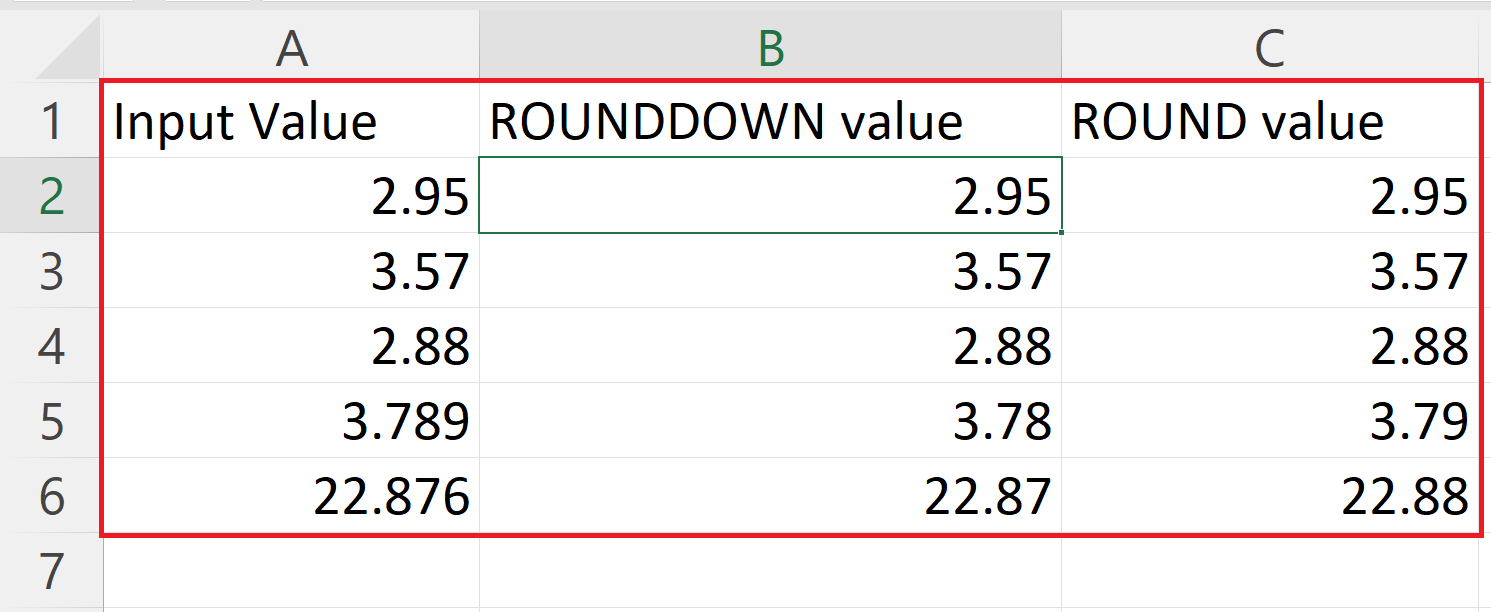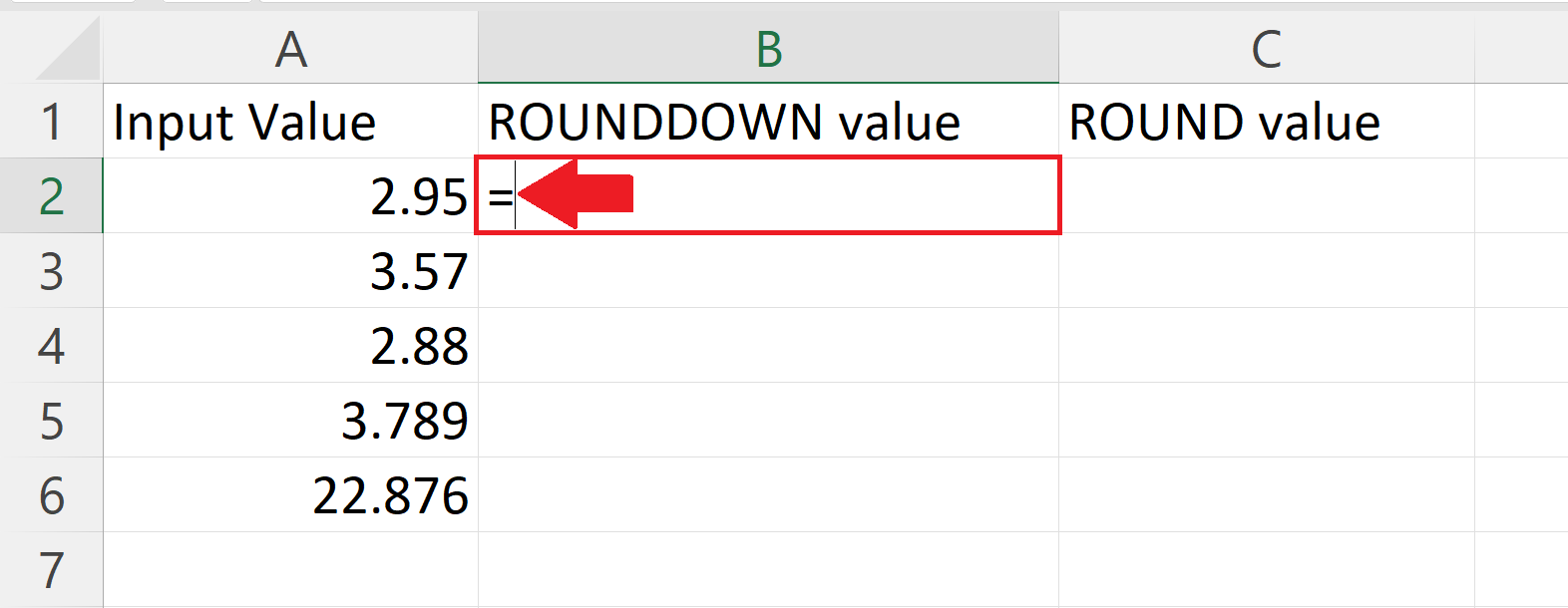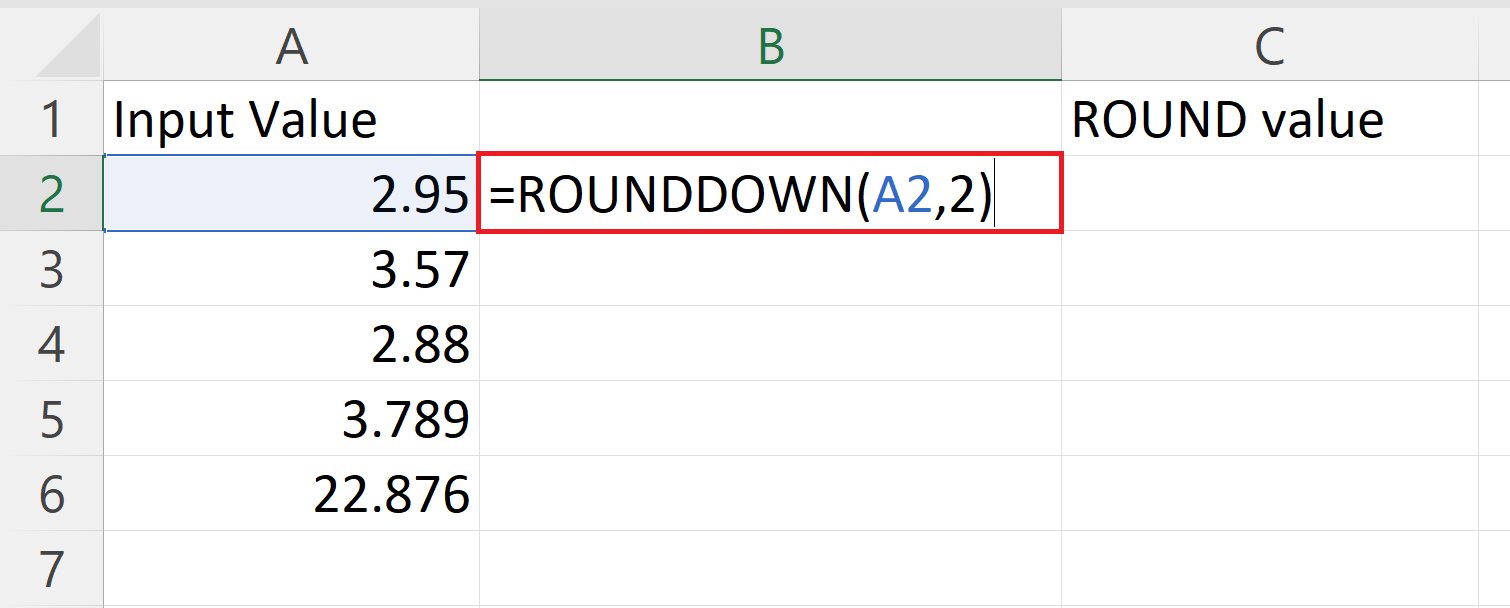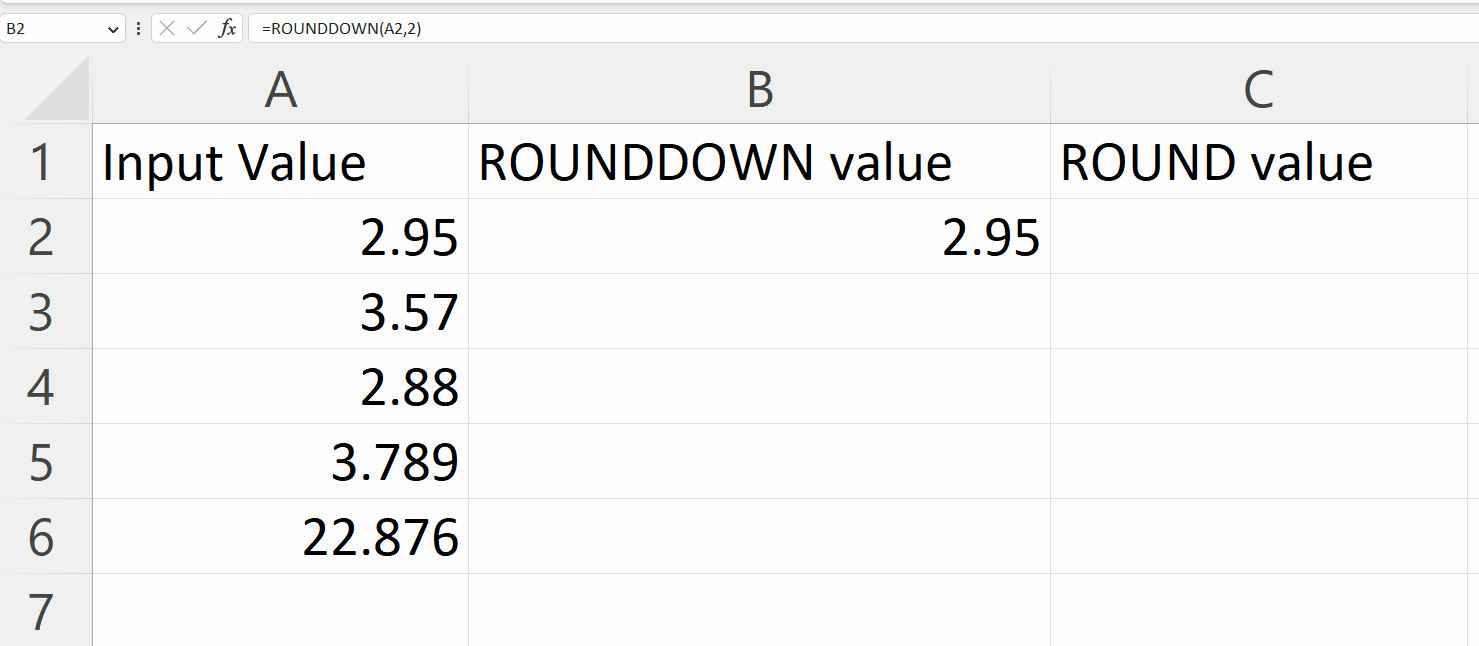# How to use ROUNDDOWN function in Microsoft Excel

In this tutorial we will learn how to use the ROUNDDOWN function in Microsoft Excel. The ROUNDDOWN function differs from the standard ROUND function in that it rounds a number down rather than up. For example, if a number 2.95 is given as an argument to both the ROUND and ROUNDDOWN functions, the ROUND function will return 3, while the ROUNDDOWN function will return 2.9.Microsoft Excel is a powerful spreadsheet software developed by Microsoft Corporation and is part of the Microsoft Office suite. It is widely used for data analysis, data management, and data presentation. Excel provides a user-friendly interface with a variety of features and tools that allow users to manipulate and analyze data with ease. It includes built-in functions and formulas for mathematical and statistical calculations, pivot tables for data summaries and grouping, and chart creation tools for visual representation of data.

### Step 1 – Select a Blank Cell– Select a targeted Blank cell where you want to ROUND DOWN a number.

### Step 2 – Place an Equals Sign### Step 3 – Use the ROUNDDOWN function– The syntax of ROUNDDOWN function is
ROUNDDOWN(A2,2)
– Where the first argument i.e. A2 is the cell containing the number to be rounded.
– The second argument i.e. 2 is an optional argument that specifies the number of decimal places to round down to.

### Step 4 – Press the Enter Key and Apply the ROUNDDOWN function to Each Row– Press the Enter key to get the results.
– Use the “handle select” and “ drag and drop” method and apply the ROUNDDOWN function to each row.

### Step 5 – Comparing ROUNDDOWN function with ROUND function– By comparing the outputs of both the ROUNDDOWN function and the ROUND function we can conclude that the ROUNDDOWN is an opposite function to the ROUND function.